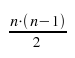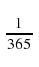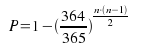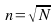## 07 April 2012According to the mathematical theory, if we put 23 people in a room there is around a 50% chance that 2 of them have the same birthday.If instead of 23 there are 75 people the probability rises  to 99.9%. Be aware that we are not talking about someone having the same birthday as us (in this case we would be imposing a condition of a specific date which is a stronger constraint), but any two people agree on her birthday (may be any date).

The mathematics behind this can be complex if you have not studied anything about probability, but we will try to explain them. To begin we must know how many possible pairings can occur in a room ofFor example, in a room with 23 people there are 253 possible pairs.
The probability that one partner has the same birthday is:Its complement is the probability that one partner does NOT have the same birthday:The probability that there is no pair with a matching birthday is:Since the base is less than 1 is likely to decrease with the increasing of the number of people in the sample. Said in another way: the more people the harder it is not to find a coincidence with the birthday. Known that, the probability (P) that we DO have a match is complementary to the above, ie:If we give the value P 0.5 (limit beyond which the chances of finding a match are placed on our side) and solve for n, we get exactly 23.

So far the theory, however it should be noted (for those who would excel at a party) that the practice is quite different since our birth dates for quite some time ceased to be equally likely because doctors increasingly scheduled births more often and not scheduled for the weekend, breaking the equiprobability!.

But the interest of the result we have obtained is not limited to the story in a meeting but we will see if we generalize the formula that has interesting applications in the field of computer security, among others. Thus, if we call p the probability that one partner will pass something and p (add a top bar) to its complement, the probability that this couple don't do what we are evaluating, then we have that  the above generalized formula is:Thus P is the probability of an event when the number of elements in the sample base is n.If we solve the formula n is as follows:For the birthday example we would like to find the number n of people from which P>0.5. To find out that we would sustituteP by 0.5, and p (with bar above) for 364/365 and would see that n is actually 23.

But lets see a more interesting example taken from the world of computer security. Suppose we are at job and a seller comes to tell us the wonders of his facial recognition system. The stuff would be placed at the entrance of the building and would replace the classic sign machine, which would eliminate the queues when the 5,000 employees of our organization arrived each morning. The commercial tells us quite self confident that the machine itself has a probability of confusing two people of about 1 in 1,000,000 (ie a probability of success of 0.999999), so he would be astonished when we reject his system for insecure . Why is it unsafe?, Because if we apply the above data to formula (taking into account that p (bar) is 0.999999) we see that the chances of error are higher than 0.5 once it has scanned 1178 employees.Put another way, there would be no way to end the morning without the little machine to make a mistake.

An useful tip is, when dealing with large numbers we can approximate the general formula with:Where N is the inverse of p (probability of occurrence with a single pair of elements). In the case of N would be 365 birthday, so that the approximation would n = 19.10 and in the case of face recognition N would be 1,000,000 and would obtain an approximation of n = 1000.

This approach is widely used in the world of cryptography in which the birthday paradox is very much in mind. For example, suppose we have a table with an encrypted password hash algorithm of 64-bit and we're calm because we believe that a dictionary attack would have to do 2 ^ 64 attempts before giving our password ... but no, nothing is further from reality as the birthday paradox shows that the attacker will surely our password when you've done little more than 2 ^ 32 attempts.

As you can see from the above, the birthday paradox transcends the merely anecdotal becoming an important tool for any security engineer.